Angle between lines

Calculate the angle between these two lines:Result

α =  45 °

Solution:Leave us a comment of example and its solution (i.e. if it is still somewhat unclear...):Be the first to comment!To solve this verbal math problem are needed these knowledge from mathematics:

For Basic calculations in analytic geometry is helpful line slope calculator. From coordinates of two points in the plane it calculate slope, normal and parametric line equation(s), slope, directional angle, direction vector, the length of segment, intersections the coordinate axes etc. Most natural application of trigonometry and trigonometric functions is a calculation of the triangles. Common and less common calculations of different types of triangles offers our triangle calculator. Word trigonometry comes from Greek and literally means triangle calculation.

Next similar examples:

1. Scalar dot productCalculate u.v if |u| = 5, |v| = 2 and when angle between the vectors u, v is: a) 60° b) 45° c) 120°
2. AngleA straight line p given by the equation ?. Calculate the size of angle in degrees between line p and y-axis.
3. PyramidPyramid has a base a = 5cm and height in v = 8 cm. a) calculate angle between plane ABV and base plane b) calculate angle between opposite side edges.
4. The angle of lines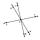Calculate the angle of two lines y=x-21 and y=-2x+14
5. BevelI have bevel in the ratio 1:6. What is the angle and how do I calculate it?
6. CotangentIf the angle α is acute, and cotg α = 1/3. Determine the value of sin α, cos α, tg α.
7. Goniometric form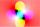Determine goniometric form of a complex number ?.On a straight stretch of road is marked 12 percent drop. What angle makes the direction of the road with the horizontal plane?
9. SlopeWhat is the slope of a line with an inclination -221°?
10. Reference angleFind the reference angle of each angle:
11. LineIt is true that the lines that do not intersect are parallel?
12. Theorem proveWe want to prove the sentence: If the natural number n is divisible by six, then n is divisible by three. From what assumption we started?
13. Parametric equationFind the parametric equation of a line with y-intercept (0,-4) and a slope of -2.
14. Points collinear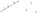Show that the point A(-1,3), B(3,2), C(11,0) are col-linear.
15. LineLine p passing through A[-10, 6] and has direction vector v=(3, 2). Is point B[7, 30] on the line p?
16. Symmetry by plane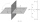Determine the coordinates of a image of point A (3, -4, -6) at a symmetry that is determined by the plane x-y-4z-13 = 0
17. Perpendicular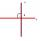Determine the slope of the line perpendicular to the line p: y = -x +4.